# Problem 2. (50 points) a) Using nodal analysis find the matrix Y and 1 of the...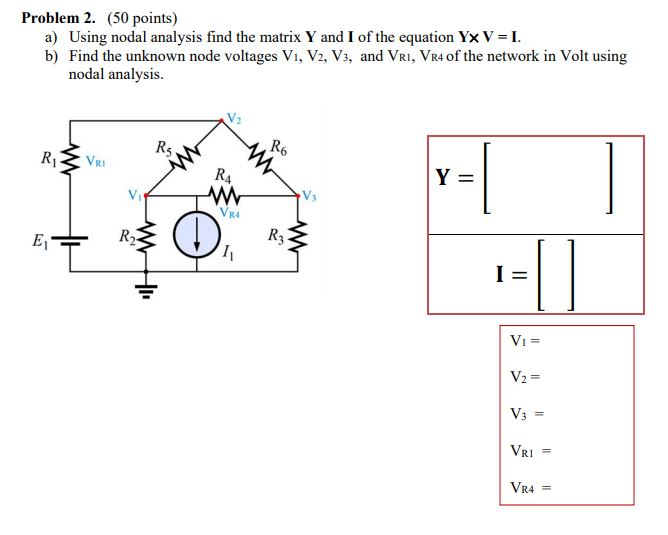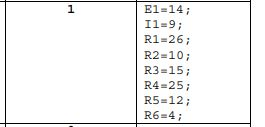Problem 2. (50 points) a) Using nodal analysis find the matrix Y and 1 of the equation Y× V-1. b) Find the unknown node voltages Vı, V2, Vs, and VRI, VR4 of the network in Volt using nodal analysis. R5 R6 RI R4 VRA VRI
4;60552; 19211214 3456 EIRRRRRR 2 1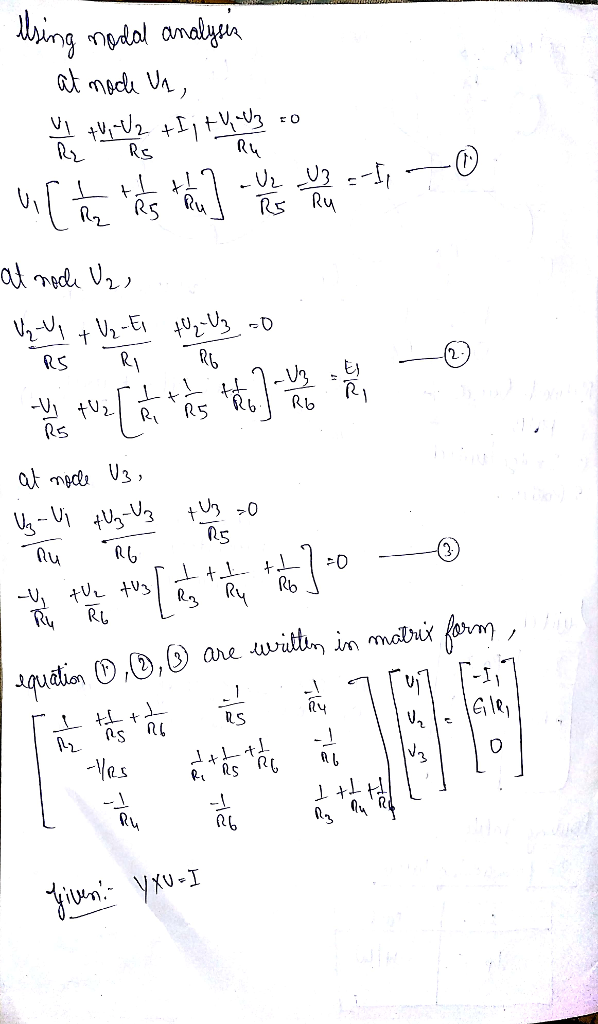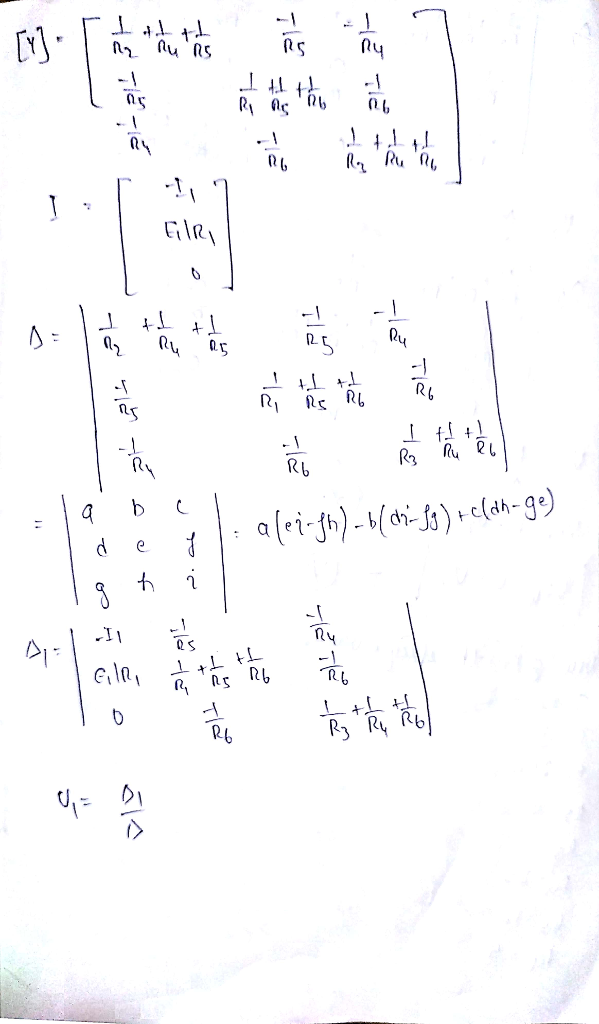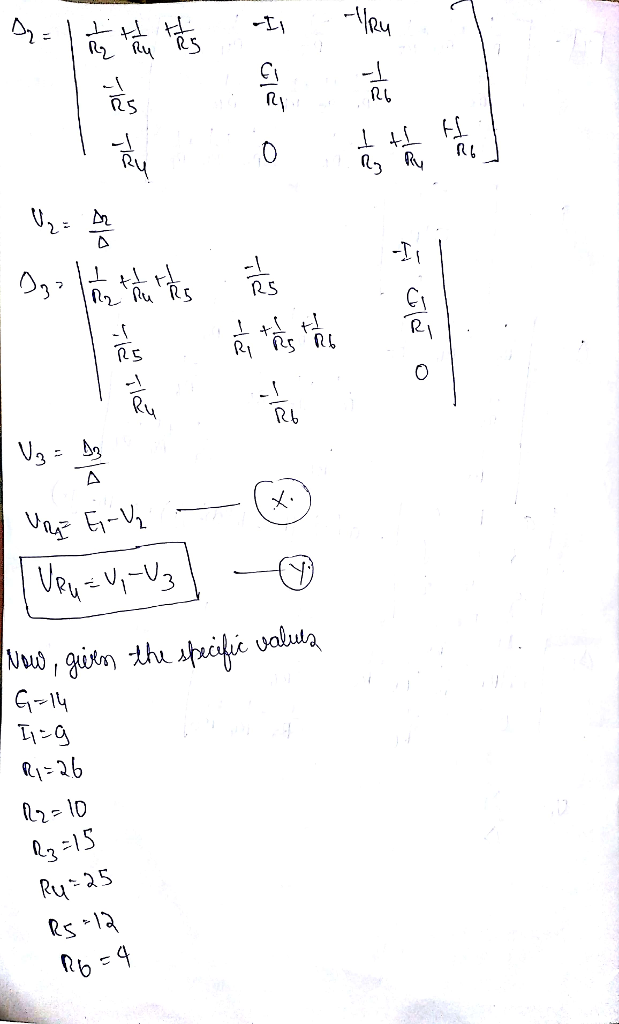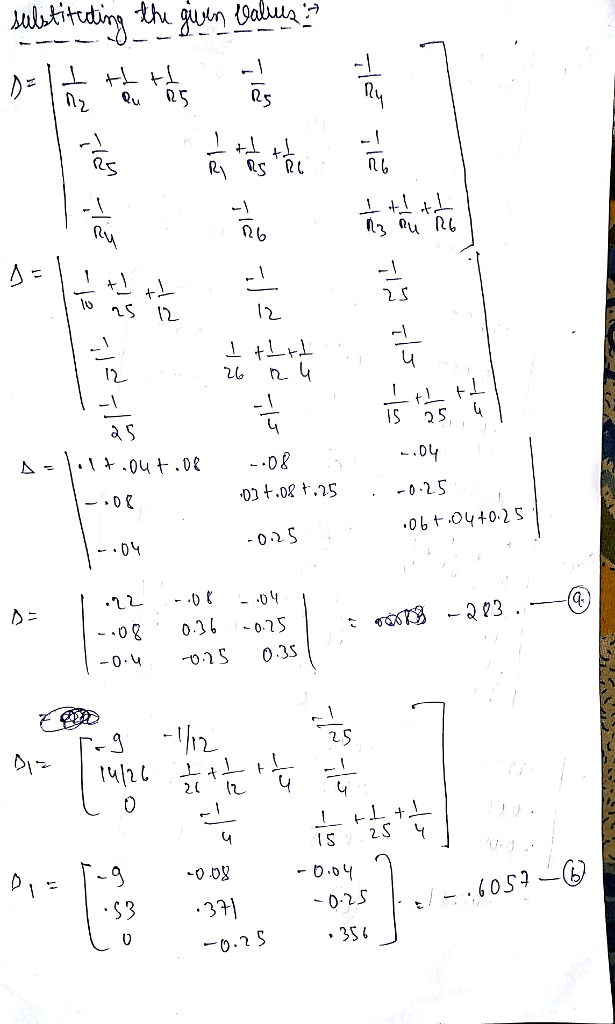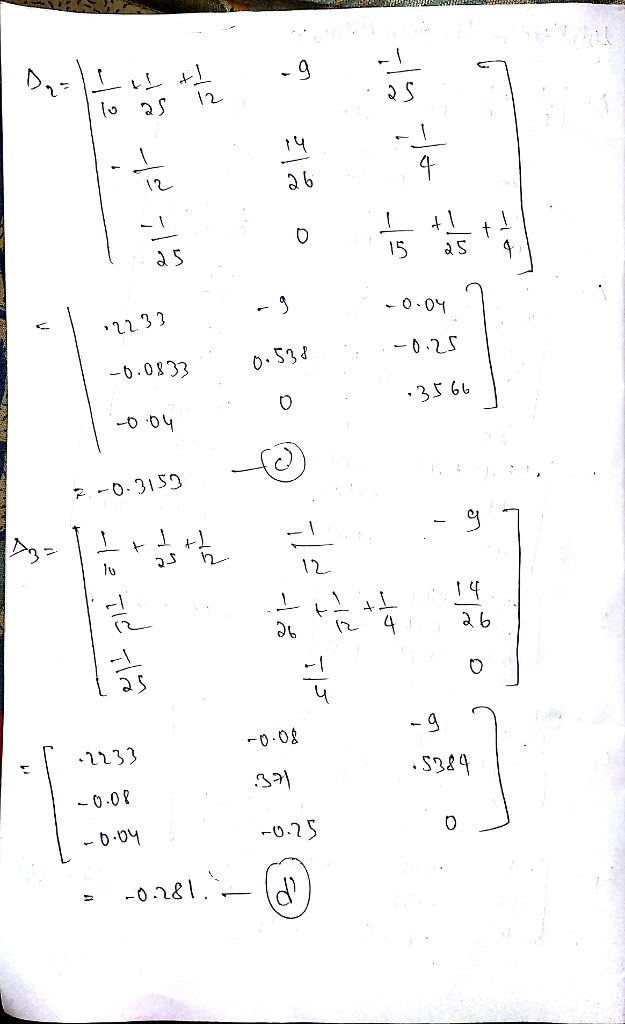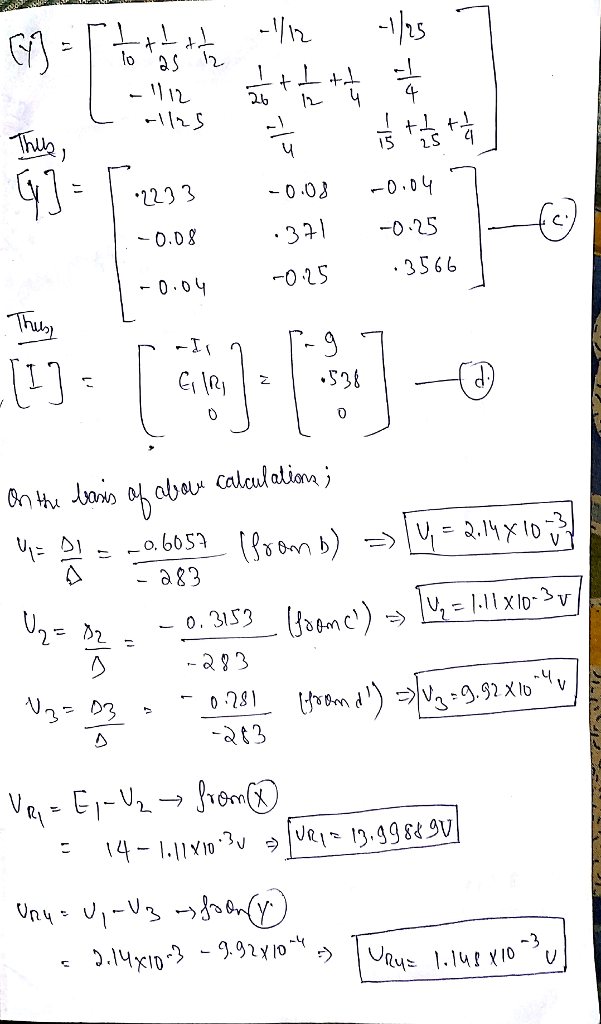#### Earn Coin

Coins can be redeemed for fabulous gifts.

Similar Homework Help Questions
• ### Problem 2. (50 points) a) Using nodal analysis (format approach) find the matrix Y and l...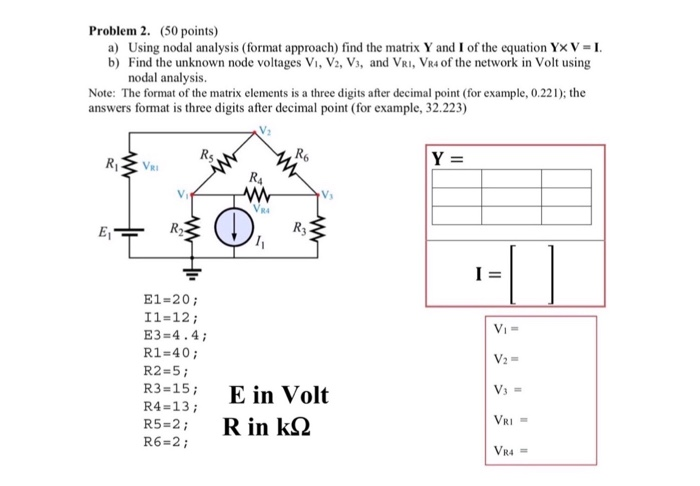Problem 2. (50 points) a) Using nodal analysis (format approach) find the matrix Y and l of the equation Y× V-I b) Find the unknown node voltages Vi, V2, Vs, and VRI, VR4 of the network in Volt using nodal analysis. Note: The format of the matrix elements is a three digits after decimal point (for example, 0.221); the answers format is three digits after decimal point (for example, 32.223) RI R4 Vi R4 R2 E1-20; I1-12 E3-4.4 R1-40 R2-5...

• ### Problem 1. (50 points) a) Using mesh analysis find the matrix R and E of the...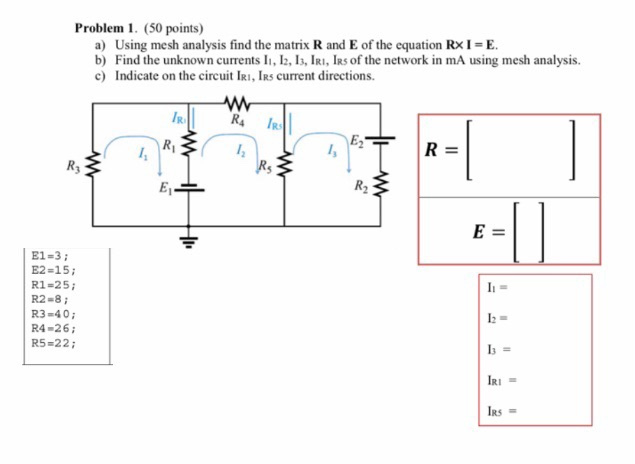Problem 1. (50 points) a) Using mesh analysis find the matrix R and E of the equation RxI-E b) Find the unknown currents ll, 12, 13, IRI, İR5of the network in mA using mesh analysis. c) Indicate on the circuit IRI, IRs current directions. R, IR E1-3 E2-15; R1-25 R2-8; R3-40 R4-26i R5-22 RS

• ### 812 7V 8A 41 S12 Perform Nodal Analysis on the circuit above and setup the matrix...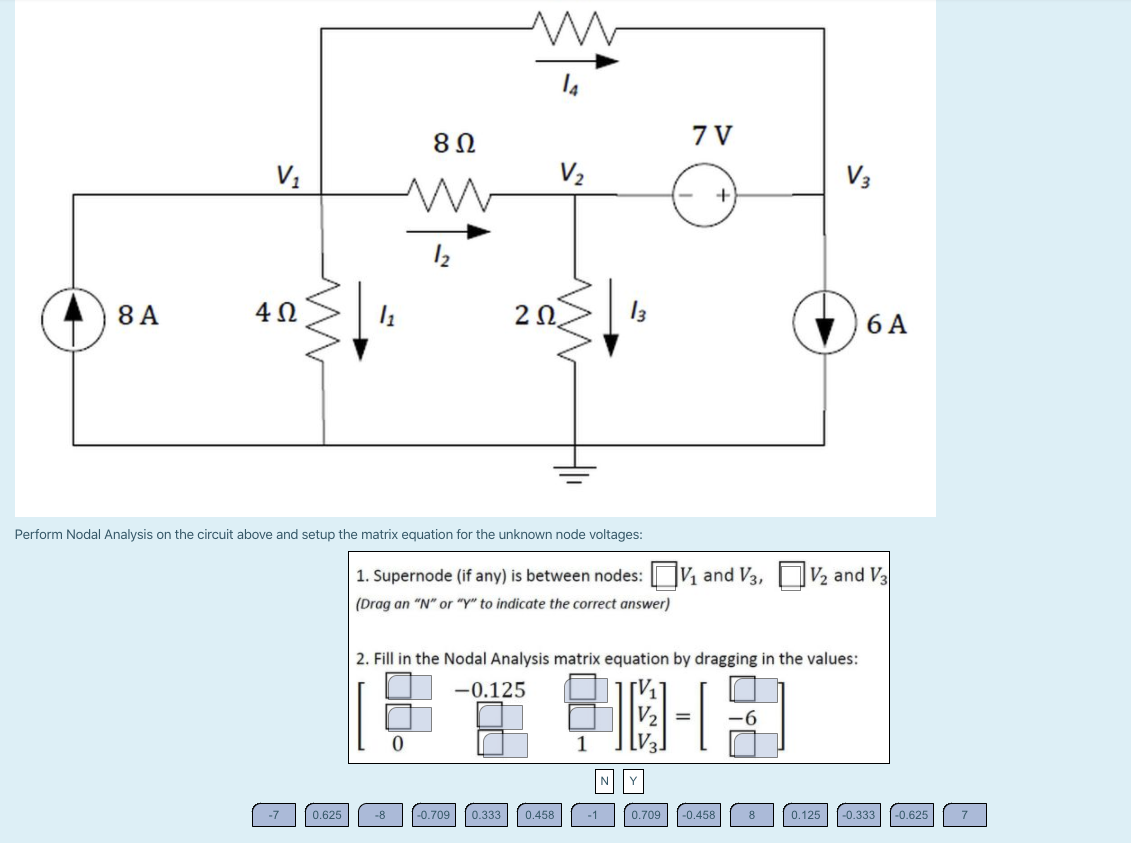812 7V 8A 41 S12 Perform Nodal Analysis on the circuit above and setup the matrix equation for the unknown node voltages: 1. Supernode (if any) is between nodes: and V3, V2 and V3 (Drag an "N" or "Y" to indicate the correct answer) 2. Fill in the Nodal Analysis matrix equation by dragging in the values: ( -100.625 -3 0.709 0.38 0.458 NY 0.709] (0.458 (0.125) (0.38) (10.525

• ### Problem 2. Find the unknown node voltages of the network using nodal analysis. 2a). (25 points)...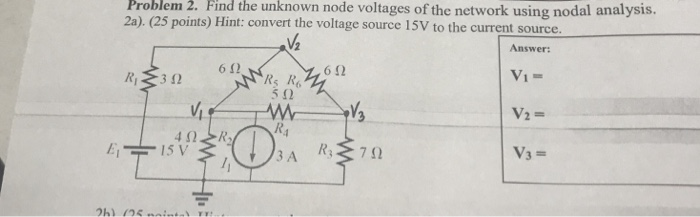Problem 2. Find the unknown node voltages of the network using nodal analysis. 2a). (25 points) Hint: convert the voltage source 15V to the current source points) Hint: convert the voltage source 15V to thec Answer: 612 612 Vi= Rs R 5 12 15 V 3A 7

• ### Not yet answered Points out of 20.00 312 Flag question Finish attempt 812 7V Perform Nodal...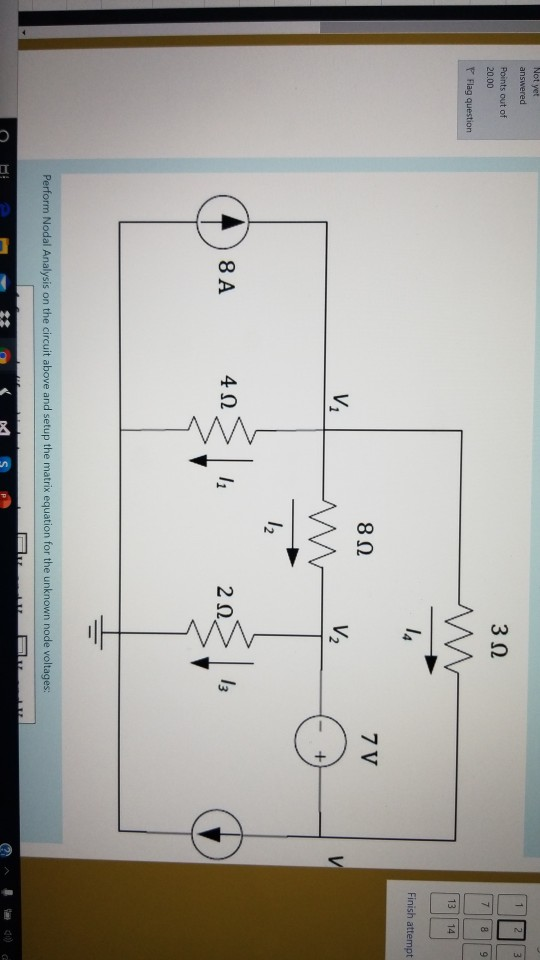Not yet answered Points out of 20.00 312 Flag question Finish attempt 812 7V Perform Nodal Analysis on the circuit above and setup the matrix equation for the unknown node voltages: - ** S S P Perform Nodal Analysis on the circuit above and setup the matrix equation for the unknown node voltages: 1. Supernode (if any) is between nodes: (Drag an "N" or "Y" to indicate the correct answer) V. and V3, V2 and V3 2. Fill in the...

• ### Find the node voltages v1, v, and v2. Use nodal analysis. R3 R 2 17 3...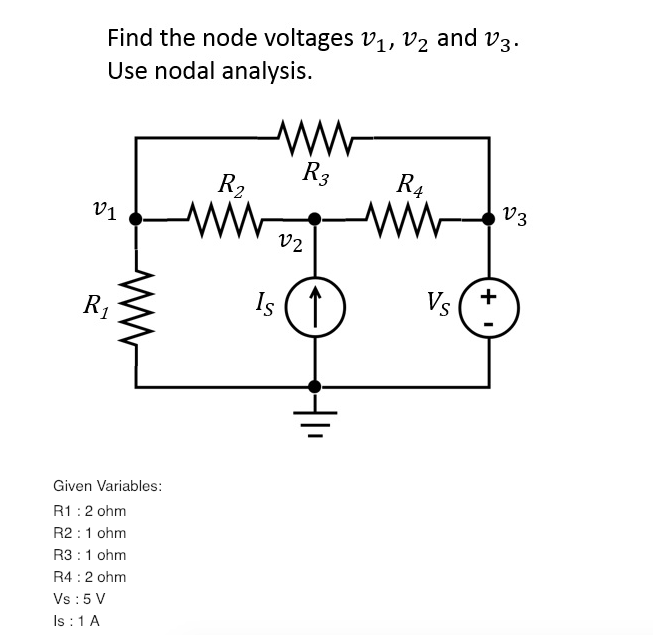Find the node voltages v1, v, and v2. Use nodal analysis. R3 R 2 17 3 1 2 Given Variables: R1:2 ohm R2 1 ohm R3 1 ohm R4 2 ohm Vs:5 V Is : 1 A

• ### just multisim 2. (20 pts) Use Nodal Analysis to determine Node Voltages Vi and V2 12...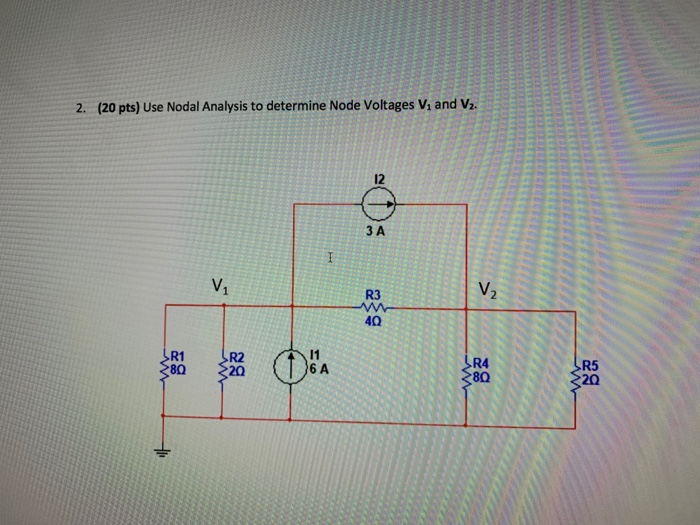just multisim 2. (20 pts) Use Nodal Analysis to determine Node Voltages Vi and V2 12 ЗА V. R3 40 R1 R4 80 R5 20 6 A 20

• ### How do I solve this ? 2. [15 points] For a circuit below, find Voltages and...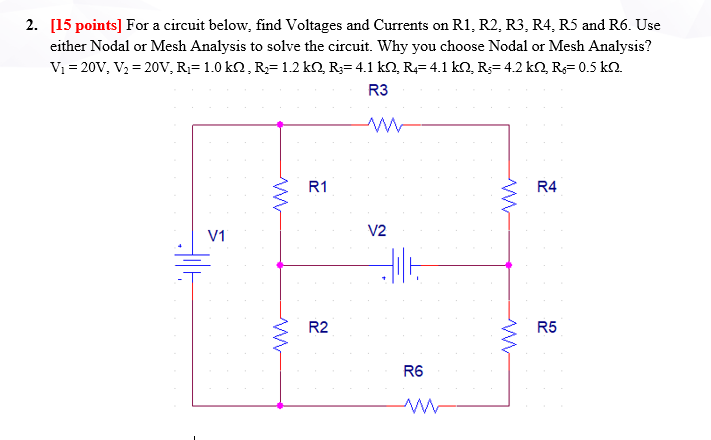How do I solve this ? 2. [15 points] For a circuit below, find Voltages and Currents on R1, R2, R3, R4, R5 and R6. Use either Nodal or Mesh Analysis to solve the circuit. Why you choose Nodal or Mesh Analysis? Vi = 20V, V3 = 20V, R;= 1.0 k1, R= 1.2 k1, R;= 4.1 kN, R = 4.1 kN, R=4.2 kN, R=0.5 k12. R3 R1 R4 V1 V2 HE Hilt R2 R5 R6

• ### 1) Find the node voltages v1, v2 and v3 using nodal analysis method. m OH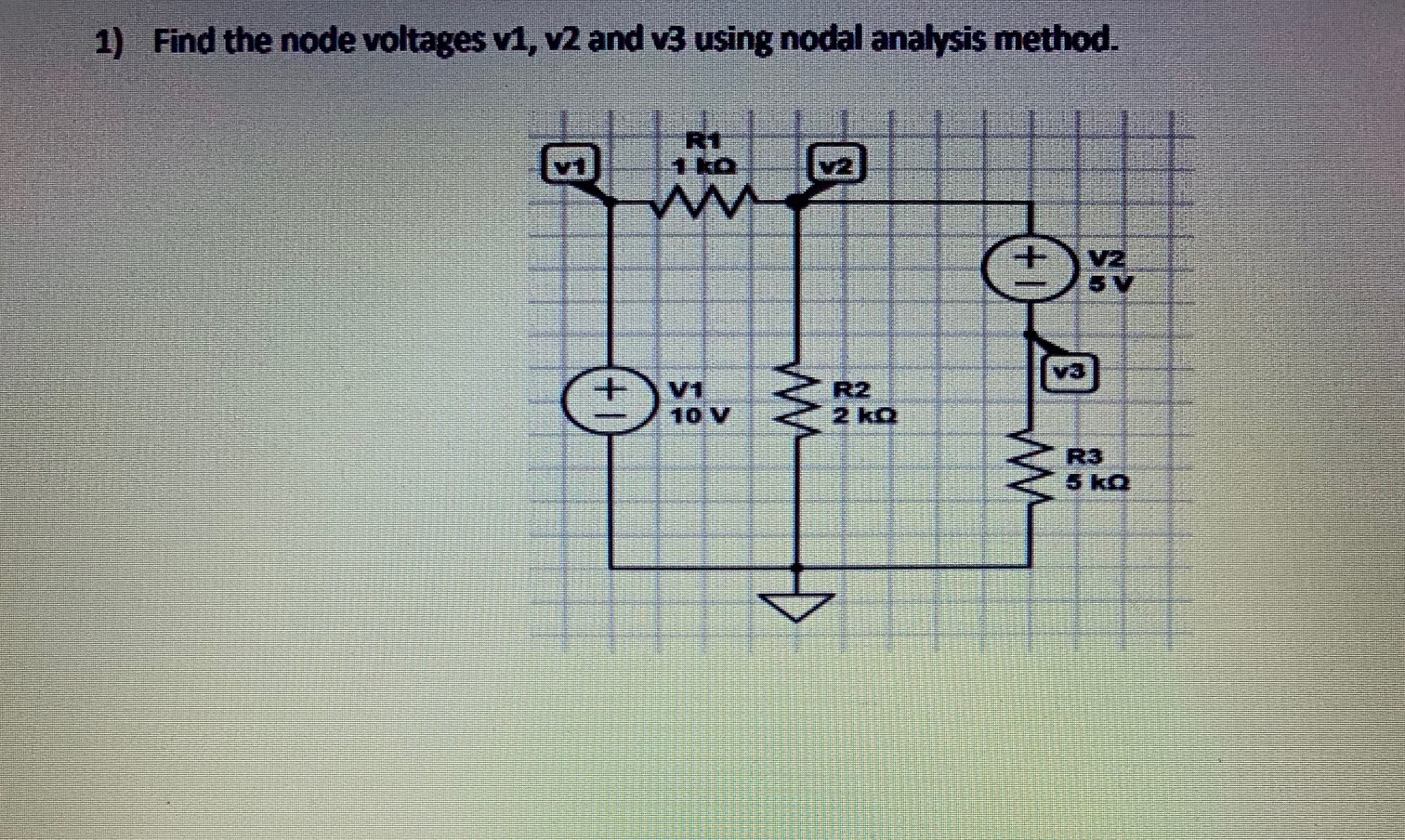1) Find the node voltages v1, v2 and v3 using nodal analysis method. m OH

• ### Using Nodal Analysis Assessment Problem 1. Determine the unknown node voltages for the following circuit, using...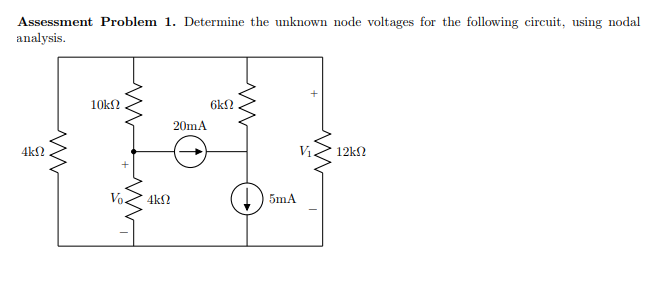Using Nodal Analysis Assessment Problem 1. Determine the unknown node voltages for the following circuit, using nodal analysis 20mA 5mA